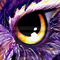# How to join multiple where() with ORnetteman
Member | 99
+
0
-

Hi,

the getSql() of

``````\$query = \$this->database->table('tableName');
\$query->select('id');
\$query->where('column1 = ? AND column2 = ?', 1, 2);
\$query->where('column3 = ?', 3);``````

is

“SELECT `id` FROM `tableName` WHERE (`column1` = ? AND `column2` = ?) AND (`column3` = ?)”

but I need to change it to

“SELECT `id` FROM `tableName` WHERE (`column1` = ? AND `column2` = ?) OR (`column3` = ?)”

Is it possible using the Database explorer?

(I need to use multiple ->where() because they will be added by a foreach())

ThanksOndřej Kubíček
Member | 494
+
0
-

i think it is not possible with this simple way.
multiple where or whereOr conditions are connected with the AND operator always.netteman
Member | 99
+
0
-

I decided to modify the SqlBuilder

``````namespace Nette\Database\Table;

class SqlBuilder
{
use Nette\SmartObject;

/** @var boolean my new property */
public \$joinWheresWithOr = FALSE;

/*code*/

/**
* Modified method buildConditions()
* @return string
*/
protected function buildConditions()
{
if(\$this->joinWheresWithOr === TRUE){
\$this->joinWheresWithOr = FALSE;
return \$this->where ? ' WHERE (' . implode(') OR (', \$this->where) . ')' : '';
}

return \$this->where ? ' WHERE (' . implode(') AND (', \$this->where) . ')' : '';
}``````

I iterate over selected rows in the presenter so I can use:

``````\$query = \$this->database->table('tableName');
\$query->select('id');
\$query->where('column1 = ? AND column2 = ?', 1, 2);
\$query->where('column3 = ?', 3);
\$query->getSqlBuilder()->joinWheresWithOr = TRUE;

foreach(\$query as \$row){
/*do stuff*/
}``````

And now the sql query looks like “SELECT id FROM tableName WHERE (column1 = ? AND column2 = ?) OR (column3 = ?)”GEpic
Member | 560
+
0
-

What about `whereOr` (doc here | api here)?

Last edited by GEpic (2018-05-24 22:36)Ondřej Kubíček
Member | 494
+
0
-

GEpic wrote:

What about `whereOr` (doc here | api here)?

as I wrote above. In docs there is written: Multiple where or whereOr conditions are connected with the AND operator.
For him it is not solution, whereOr is for connection several params with OR but not for multiple where or whereOr

Last edited by Ondřej Kubíček (2018-05-24 23:04)GEpic
Member | 560
+
0
-

And what about using only whereOr and use AND inside in brackets (like `->whereOr("(column = ? AND column2 = ?", \$c1, \$c2)`, should it work?

Last edited by GEpic (2018-05-25 14:58)